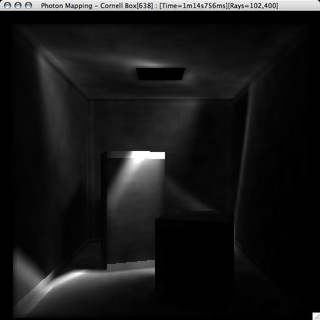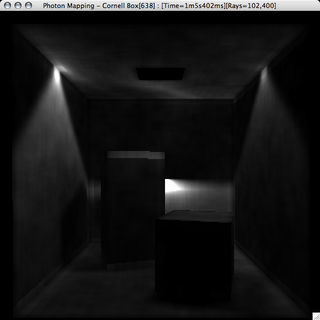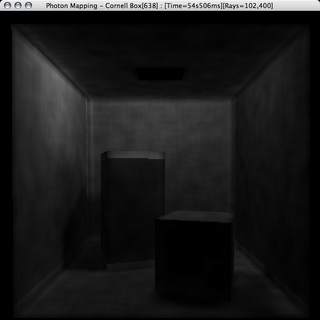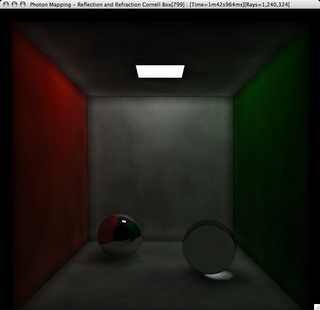# Photon Mapping

Photon mapping is a way to do illumination in 3D graphics. This is my computer graphics project from college.

# Photon Mapping: What is it?

Okay, really quick, photon mapping is where we cast photons from the light out into the scene we are drawing and store their interactions with the geometry in a photon map. Then we to a raytrace on the scene, but when we do our lighting calculations we use the information in the photon map as well as direct illumination. This gives us indirect illumination, and with the addition of a second photon map we can do good, cheap caustics. For more info check wikipedia. This is an extension of my previous project which is a ray tracer, you can read about it on its blog.

# Quads as Area Lights

A bit out of order, but this post will deal with my work on using quads for area lights. Okay, here's the deal, how do you pick a random point from the interior of a quadrilateral and ensure that the distribution is as uniform as possible? Good question ehh? My math prof thinks so too. We discussed several methods. We are doing this because we need to emit photons from the surface of the light.

The following pictures of distributions are plotted with 2500 points.

###Its easy to select a point inside the parallelogram defined by a triangle, so divide the quad into four triangles, pick a point inside each corresponding parallelogram and average them together. Nice and simple, but it creates a distribution that is obviously not uniform, it shows a strong bias for the center of the quad. To be fair, this was of my own invention. (The red, green, yellow, and cyan dots are from the four triangles, the blue are the actually points.)

###This method my math prof and I came up with. Since the definition of convex is that any two points picked on or in a convex shape, the line segment connecting those points is entirely contained in or on the shape. So we pick two random points along the edges of the quad, we then pick a random point along the line segment that connects those two points. This gives us the following distribution, which looks decent, but you can notice a bias for the edges. Which comes from the fact that the two edge points might fall on the same edge.

### Method C: Edge-Edge-Line Method - Second Try

This is the same method as above, Method B, but we add the restriction that the two points on the edges are not allowed to ever be on the same edge. We get this distribution which looks a lot better. Here are a couple more images. These are 20,000 points apiece. You can see that both Methods B and C work fairly well, but I'll choose Method C for my work.

### Method C:# Success! Atleast with GIThis has been an incredibly annoying project. Granted it stems from the fact that I didn't have all the resources I needed as far as the minute detials behind programming the ray tracer. I didn't have Jensen's book Realistic Image Synthesis Using Photon Mapping, which supposedly is a pretty decent book, and most importantly has functioning code in the back. The biggest problem I had was that everyone explained the generalities of the algorithem, but not the depths of the implementation, and no one posted their code up, which I will do on the completion of my project, in hopes that someone else won't go through what I did.

There were a fair number of more specfic problems with my implementation of a photon-mapper. One of which were my photon lights, and my seemingly inability to get the photons to cast in the correct directions. This has to be a side effect of the algorithem used for picking a random-point on a sphere, but as why I had so much trouble is unbeknowst to me. Here are three images showing a grays-scale direct visualization of the photon map, i.e. the bright the area, the more photons landed neat there.

This first picture is from my first method of picking a point on a hemisphere to aim the photons from the light. I have no idea what is up with the focusing and the voids, it completely baffles me.Here is a slightly better image, the photons are shooting in more reasonible directions in reasonibl concentrations, but still its not good enough, things just are quite right.

Finally, after moving to an entirely new method of picking a point on a sphere I get this, which looks great!For those of you who care, here are my methods for generating points on a unit sphere. This first one is just not working for me:

```        double x1 = 0, x2 = 0, x12 = 0, x22 = 0;
do
{
x1 = RAND.uniform( -1, 1 );
x2 = RAND.uniform( -1, 1 );
x12 = x1 * x1;
x22 = x2 * x2;
}
while( x12 + x22 >= 1 );

double x = 2 * x1 * Math.sqrt( 1 - x12 - x22 );
double y = 2 * x2 * Math.sqrt( 1 - x12 - x22 );
double z = 1 - 2 * ( x1 + x2 );

// Stupid co-ordinate frame differences
Vector3d vec = new Vector3d( x, z, y );
return vec;
```

This second one works great for me, so its the one I'm using now:

```        double z = randGen.uniform( -1, 1 );
double t = randGen.uniform( 0, 2.0 * Math.PI );
double w = Math.sqrt( 1 - z * z );
double x = w * Math.cos( t );
double y = w * Math.sin( t );
Vector3d vec = new Vector3d( x, y, z );

// If it is on the other side of the normal, negate the point
if( normal.dot( vec ) < 0 )
{
vec.negate();
}

return vec;```

# Multi-Threading and Storing Photon Maps

Well, since I'm running with a Core Duo I decided to implement multi-threading for the photon scattering step since thats where 90% of the time is spent. It works great and was very simple to do, required very little restructuring of my code.

And to add another speed book I made the photon map serializable so that I can save and load them to disk. It saves some time, but the deserializing process is

slow

and I mean slow. But it helps.

More to come later, this was just a quick note between renders.

# Global Illumination Irradiance Estimation and Photon Prunning

Photon irradiance is estimated using a spherical area. However this alone will produce incorrect results. Check out this image, notice banding along all the surfaces that meet at 90 degrees. This is because just grabbing all the photons within a sphere gets photons from both surfaces, when really the photons from surfaces that are orthogonal to each other should not contribute to the lighting.

If we add a simple check looking at the angles between the photons incident direction and the normal of the current surface and throwing out the ones that have angles greater than 90 degrees we notice a great reduction in banding.

This still doesn't fix everything, the last thing we will do is scale the contribution of the photon by the cosine of the angle between the photons original incident surface's normal and the normal of the current surface. This makes the best looking image

Up until now I have just been using the original search radius from the kd-tree searching to use in the irradiance estimation steps. But someone mentioned and flaw in the images, the dark edges along boxes, and I thought that perhaps the radius was wrong. For reference here is an image with that uses the search radius in the irradiance estimation.

So after thinking about the radius and how the photons are gathered and used I decided to recalculate the radius that would actually be used in the irradiance estimation by calculating the radius of the sphere containing the photons that we would actually be using for the irradiance estimation, this would shrink the sphere if we threw out alot of photons because of pruning steps. It produces this image, which looks better and is in my mind more accurate.

One thing that I think is an interesting side effect is the new banding that shows up on the walls, especially the back one. I'm not sure what is causing it and I don't know if its better or worse than the standard blotchy noise, as far as appearance goes. Another thing to note is the lighting is better, we don't have the super bright spots on the walls anymore.

# CausticsI'm having a bit of trouble getting caustics to look right, I'm not sure what the problem is. My emmission code looks good an the results of the photon intersections appear to be accurate, as in they fall within cones aimed at the reflective and transparent objects. The algorithem for picking a point in a cone is pretty cool, its of my own design. If we need three pieces of information about the cone, the starting point or tip, the center of the base, and the radius at the base. To pick a direction that lies with in the cone's volume we first pick a point on the unit hemisphere oriented along the ray from the start to the end of the cone. We then scale that point by the radius of the cone and add that scaled point onto the end point, this creates a point on a hemisphere glued to the end of the cone. Our direction is then the unit vector from the start to this new point.

Here's a picture of the caustics as they stand:

# High-Res Picture

Nothing new, just a high-res picture.